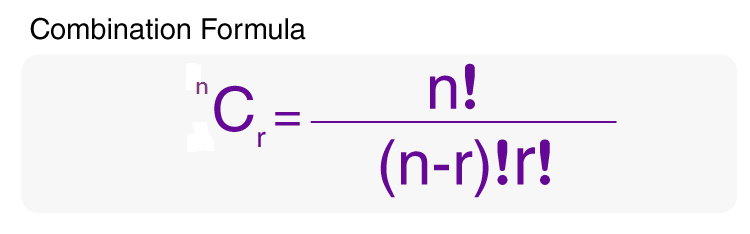# Combination Formula

The combination formula is used to find the number of ways of selecting items from a collection, such that the order of selection does not matter. In simple words, combination involves the selection of objects or things out of a larger group where order doesn’t matter.

The formula for combination helps to find the number of possible combinations that can be obtained by taking a subset of items from a larger set. It shows how many different possible subsets can be made from the larger set. It should be noted that the formula for permutation and combination are interrelated and are mentioned below.## Formula for Combination

 Combination Formula $$\begin{array}{l}^{n}C_{r} = \frac{n!}{(n-r)!r!}\end{array}$$ Combination Formula Using Permutation C(n, r) = P(n,r)/ r!

### Notations in nCr Formula:

• r is the size of each permutation
• n is the size of the set from which elements are permuted
• n, r are non-negative integers
• ! is the factorial operator

The combination formula shows the number of ways a sample of “r” elements can be obtained from a larger set of “n” distinguishable objects.

Also Check: N Choose K Formula

### Example Question From Combination Formula

Question 1: Father asks his son to choose 4 items from the table. If the table has 18 items to choose, how many different answers could the son give?

Solution:
Given,
r = 4 (item sub-set)
n = 18 (larger item)

Therefore, simply: find “18 Choose 4”

We know that, Combination = C(n, r) = n!/r!(n–r)!

$$\begin{array}{l}\frac{18!}{4!(18-4)!}=\frac{18!}{14!\times4!}\end{array}$$